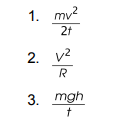# IMAT 2013 Q57 [Units of Power]

In the expressions below: g = gravitational acceleration; h = height; m = mass;
R = electrical resistance; t = time; v = velocity; V = voltage.

Which of the following expressions have units of power?A. 1 and 2 only
B. 1 only
C. 2 and 3 only
D. 1 and 3 only
E. 1, 2 and 3

1 Like

Remember that Power is the rate at which energy is transferred from one system to another. A familiar formula should be P=E/t.

Expression 3 should look the most familiar. Since GPE= mgh, mgh/t is the rate at which gravitational potential energy is transferred from one system to anther; and it is correct.

We can eliminate A and B.

You’ll notice Expression 2 is the formula for Electric Power. It derives from a combination of Ohm’s Law and another power equation. Look here:

P= VI
but also
P= I^2 R

Which means that P= \frac{V^2}{R} and that Expression 2 is correct.

We can eliminate D.

Expression 1 is also correct, as it is the rate at which kinetic energy is transferred from one system to another. Recall that K.E= 1/2mv^2. The rate of which would simply be \frac{mv^2} {2t}.

All 3 expressions are correct and E is our answer.